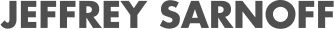# AtomicSets.jl

07/29/2021, 4:40 PM4:50 PM UTC
Red

#### Abstract:

We present `AtomicSets.jl`, a Julian framework for structured convex optimization. Algorithms for structured optimization build up a solution from a set of prescribed atoms that represent simple structures. The atoms that participate in the final solution often represent key explanatory components of a model. We use Julia's dispatch system to implement a calculus of convex sets and their functional representations that compiles to efficient machine code.

#### Description:

AtomicSets.jl was developed by Michael Friedlander, Zhenan Fan and Mia Kramer at the University of British Columbia.

We say a set is convex if, for every pair of points in the set, the line between those points is also contained in the set. This is a generalization of the notion of convex that most of us would have learned in grade school for polygons: the set doesn't have any "caves" or "dents". We similarly call a function convex if its epigraph—the set of points "above" the function if it were plotted—is convex. Some common examples of useful convex sets are the one ball—the set of all points with 1-norm less than or equal to one, and the nuclear ball—the set of all matrices that can be written as an outer product of unit norm vectors. We care about convexity because it guarantees some useful properties for optimization. For exampe: any local minimum of a convex function is also a global minimum. Combinations of these properties can give us efficient algorithms with strong convergence guarantees.

Suppose we are trying to solve a problem where our answer is a vector, and we expect it to be sparse. In general, computing with exact sparsity is difficult, but let's take a step back. To say that a vector is sparse is to say it should be constructed from a small number of coordinate vectors, each scaled by a nonnegative amount. Let's take the coordinate vectors (and their opposite sign counterparts) to be our atoms, and take their convex hull to be our domain. We now have a domain we know to be convex, which also induces the structure we want to see in our solution. The process is similar for low-rank matrices: we assume that they will be constructed from a small number of outer products, so we take the set of unit rank outer products to be our atoms.

To generalize over these atomic sets, we need more than their common properties; we need a set of common operations. The most basic of these is probably the `gauge` function. Given a set A and a point x, the gauge function answers the question "what is the smallest scale λ such that x is in λ A?" In other words, how much do we have to expand or contract A so that x is only just contained in it? If we pick our set to be the two ball, the set of points with Euclidean norm ≤ 1, our gauge function is just the Euclidean norm of the point.

Other common operations we have on atomic sets are the `expose` and `support` functions. The `expose` function gives us the atom which is most aligned with a given vector, where aligned means maximizing the dot product. Another way to imagine the operation is to take your set and your vector, and then sweep a hyperplane from the origin in the direction of the vector. The last point that the hyperplane touches on its path is the exposed point. The `support` function gives the value of the inner product which produced the exposed point.

Additionally, we define a calculus of atomic sets. We can construct atomic sets that are, for example, the Minkowski sum of other sets, a scaling of a set, or a linear map applied on a set. The `expose` operation gives us in some sense an element of the subderivative of the set, and so the usual chain rule applies. By defining this operation recursively for these compound sets, they too can be generic.

Using Julia's type system, we build representations for the sets, their atoms, and faces (collections of atoms). By writing functions using these common operations on atomic sets, Julia's dispatch system allows compiling said function for any choice of atomic set (and hence notion of sparsity). Using this construction, we present a dual method for solving min_x ½ ‖ Mx - b ‖² s.t. gauge_A(x) ≤ τ, which is generic over the choice of set A.